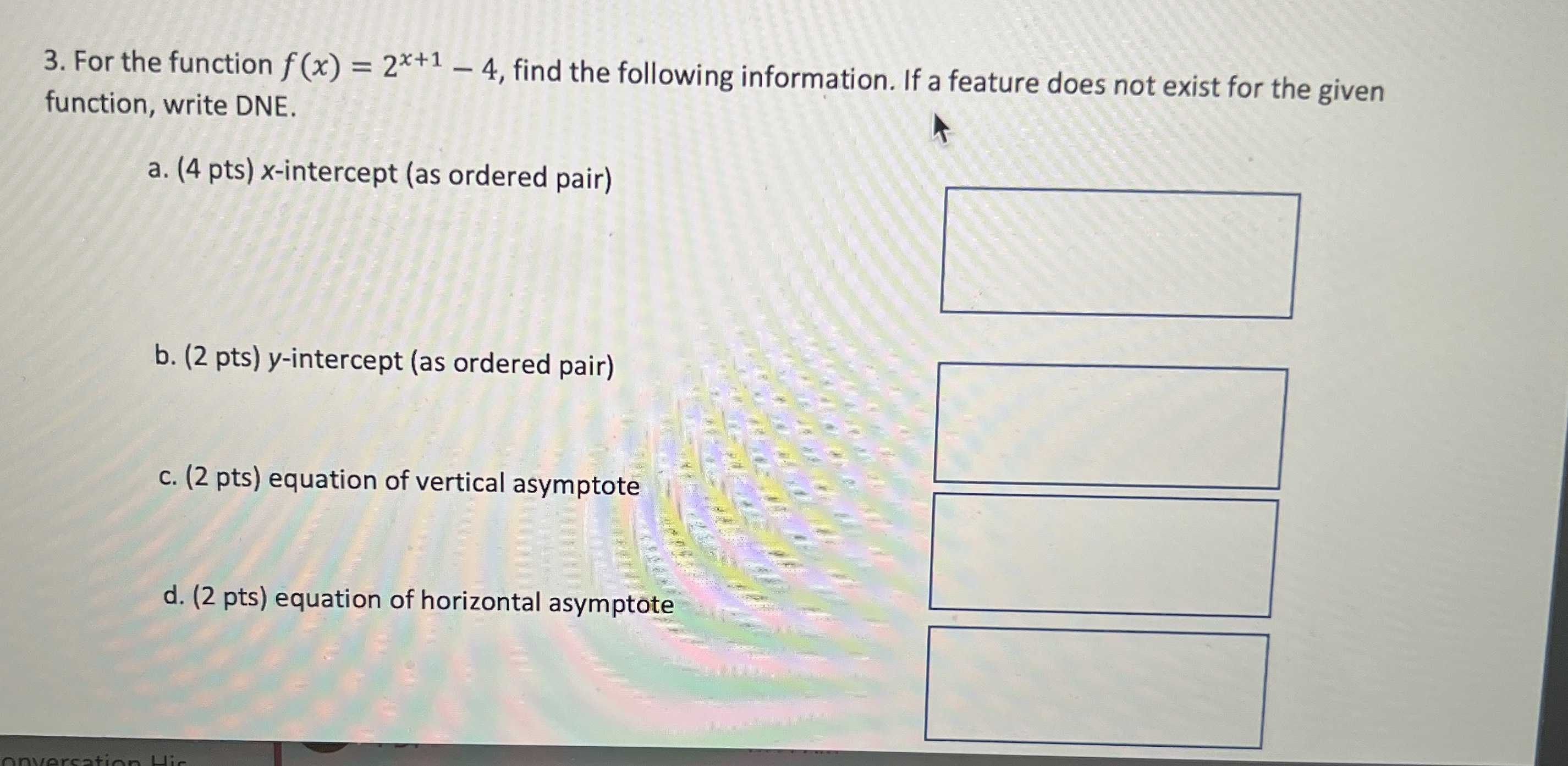### ¿Todavía tienes preguntas de matemáticas?

Pregunte a nuestros tutores expertos
Algebra
Pregunta3. For the function $$f ( x ) = 2 ^ { x + 1 } - 4$$ , find the following information. If a feature does not exist for the given function, write DNE. a. ( $$4$$ pts) $$x$$ -intercept (as ordered pair) b. ( $$2$$ pts) $$y$$ -intercept (as ordered pair) c. (2 pts) equation of vertical asymptote d. (2 pts) equation of horizontal asymptote

(a):

x-intercept(s): $$( 1,0 )$$

(b):

$$y$$ -intercept $$( s ) : ( 0 , - 2 )$$

(c):

DNE

(d):

Horizontal Asymptote: $$y = - 4$$

Solución
View full explanation on CameraMath App.